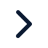Executive ProgramsWorkshopsProjectsBlogsCareersFind JobsCorporate TrainingHire from USAll Courses

Choose a category

All Courses

All Courses# Matlab program to calculate the global maxima of a stalagmite function

A genetic algorithm is a method of solving optimization problems by the concept of theory of evolution proposed by Charles Darwin. This algorithm is used to calculate the global minimum or global maximum of a function. The working of a genetic algorithm is given below  1. The algorithm randomly selects members from…

Project Details

Thanks for choosing to leave a comment. Please keep in mind that all the comments are moderated as per our comment policy, and your email will not be published for privacy reasons. Please leave a personal & meaningful conversation.

Be the first to add a comment

Flow over a cylinder

Objective:

Simulating the flow over a cylinder with different reynolds number A cylinder is designed in solidworks of the following dimensions Diameter = 40mm Length = 50mm The formula to calculate the value of velocity for different reynolds number is given as, The simulation is carried out for 3 different reynolds number Re=20,40,100,…21 Sep 2018 09:13 AM IST

Pipe flow simulation

Objective:

A pipe is designed in solidworks of the following dimensions, to perform the simulation External diameter = 50mm Internal  diameter = 48mm Length = 1000mm Thickness = 1mm The characteristics of the fluid which is flowing inside the pipe are as follows, Fluid : water Dynamic viscosity : 0.001792 kg/ms (at 0°C…05 Sep 2018 08:12 AM IST

Project -1 Parsing NASA thermodynamic data

Objective:

NASA  came up with polynomials that can be used to evaluate thermodynamic properties such as Cp, H and S using polynomials. They have also documented the co-efficients that are required to evaluate these polynomials for 1000+ species. The program that can read the high and low temperature data from the given data…03 Aug 2018 05:57 AM IST

Matlab program to calculate the global maxima of a stalagmite function

Objective:

A genetic algorithm is a method of solving optimization problems by the concept of theory of evolution proposed by Charles Darwin. This algorithm is used to calculate the global minimum or global maximum of a function. The working of a genetic algorithm is given below  1. The algorithm randomly selects members from…02 Jul 2018 09:28 AM IST

Second order ODE

Objective:

Video of moving pendulum https://youtu.be/kDixJr7sMAU   The code for solving the second order ODE, clear all close all clc %inputs b=0.5; g=9.81; l=1; m=1; %initial conditions theta_0=[0;3]; %time plots t_span = linspace(0,20,500); %solve ODE [t,results]= ode45(@(t,theta) ode_func(t,theta,b,g,l,m),t_span,theta_0);…30 Jun 2018 11:17 AM IST

Curve fitting

Objective:

Q1>Write code to fit a linear and cubic polynomial for the Cp data. Explain if your results are good or bad.  The code to fit a linear polynomial for the given cp data is given below, clear all close all clc %preparing the data cp_data = load ('data') temperature = cp_data(:,1); specific_heat = cp_data(:,2); %curve…30 Jun 2018 07:58 AM IST

Simulation of a 2R robotic arm

Objective:

%forward Kinematics animation animation of a 2r robotics arm clear all; close all; clc; %inputs l1=1; l2=0.5; theta1 = linspace (0,90,4); theta2 = linspace (0,90,4); ct=1; for i=1:length(theta1) THETA1= theta1(i); for j=1:length(theta2) THETA2 =theta2(j); %coordinates x0 = 0; y0 = 0; x1 = l1*cosd(THETA1); y1 = l1*sind(THETA1);…29 Jun 2018 04:15 AM IST

Otto Cycle PV diagram and efficiency

Objective:

Function to calculate the piston kinematics function [V] = otto_cycle_piston_kinematics(bore,stroke,con_rod,cr,start_crank,end_crank) %inputs theta = linspace(start_crank,end_crank,100); a=stroke/2; R = con_rod/a; v_swept = (pi/4)*bore^2*stroke; v_clearence = v_swept/(cr-1); %the volume of the piston can be calculated…01 Jun 2018 04:23 AM IST

Matlab program to analyze the flow over a bicycle

Objective:

% Program to calculate the drag force on a bicycle clear all close all clc %inputs %drag coefficient c_d = 0.8; %velocity m/s v= [1:40]; %density m^3/s d= 1.2; %area m^2/s a=0.1; %drag force calculation drag_force = d*a*v.^2*c_d*0.5; %plotting figure(1) plot(v,drag_force) title('Velocity (vs) Drag force') xlabel('Velocity')…01 Jun 2018 04:09 AM IST

Showing 1 of 9 projects
Try our top engineering courses, projects & workshops today!